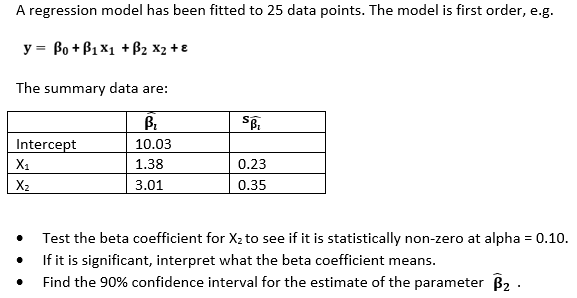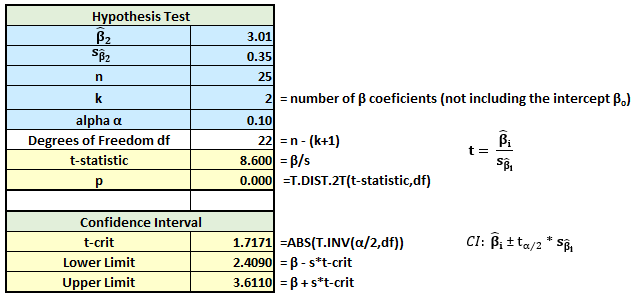# More on Multiple Regression Part 2

In the real world, we most often work with real data. And we build regression models using software such as Excel, StatCrunch, and, more recently, R. The output of those regressions give us a world of information about the regression models, enabling us to quickly determine if the overall model is statistically significant and if the individual regression coefficients are as well. And with most software, getting confidence intervals associated with the regression model is straightforward.

But in the world of academia, my students often are confronted with an inability to work on the actual data and are instead presented with partial regression output. I know the intent is to make sure students understand the nuts and bolts of regression, but my sense that could be done just as well or better with real data.

That said, I want to help my students learn about regression and get through their academic course with a satisfactory grade.

Here is a typical problem probing understanding of regression intermediates:Unfortunately, StatCrunch and other common software are not set up to do this work. So, I suggest using Excel.

First, we need to state the null and alternative. For these types of problems,
Ho: β= 0; Ha: β≠ 0.The p-value of 0.000 tells us hypothesis test is statistically significant at alpha = 0.10, and we reject the null. We conclude β2 ≠ 0. For every 1 unit change in X2, y increases 3.01 units.

The 90% confidence interval for β2 is (2.409, 3.611). In general, that means we can be 90% confident the true value of the parameter β2 being estimated lies in the interval.

You can repeat the sequence for each β being estimated.

This site uses Akismet to reduce spam. Learn how your comment data is processed.